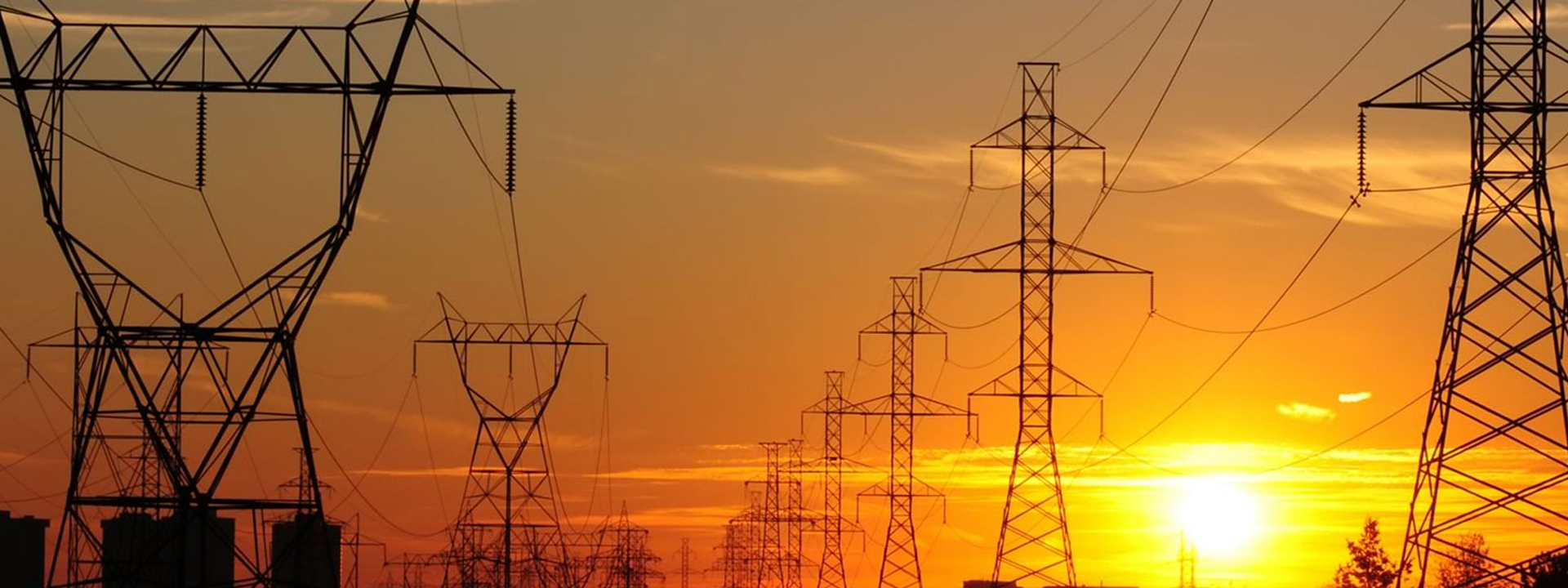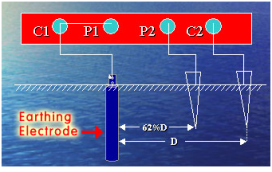••••### Measurement of Earth Resistance by use of Earth Tester:

1. For measuring soil resistivity Earth Tester is used. It is also called the âMEGGERâ.
2. It has a voltage source, a meter to measure Resistance in ohms, switches to change instrument range, Wires to connect terminal to Earth Electrode and Spikes.
3. It is measured by using Four Terminal Earth Tester Instrument. The terminals are connected by wires as in illustration
4. P=Potential Spike and C=Current Spike. The distance between the spikes may be 1M, 2M, 5M, 10M, 35M, and 50M.
5. All spikes are equidistant and in straight line to maintain electrical continuity. Take measurement in different directions.
6. Soil resistivity =2ÏLR.

7. Measurement Of Earth Resistance8. R= Value of Earth resistance in ohm.
9. Distance between the spikes in cm.
10. Earth resistance value is directly proportional to Soil resistivity value.
11. In this method earth tester terminal C1 & P1 are shorted to each other and connected to the earth electrode (pipe) under test.
12. Terminals P2 & C2 are connected to the two separate spikes driven in earth. These two spikes are kept in same line at the distance of 25 meters and 50 meters due to which there will not be mutual interference in the field of individual spikes.
13. If we rotate generator handle with specific speed we get directly earth resistance on scale
14. Spike length in the earth should not be more than 1/20th distance between two spikes.
15. Resistance must be verified by increasing or decreasing the distance between the tester electrode and the spikes by 5 meter. Normally, the length of wires should be 10 and 15 Meter or in proportion of 62% of âD'.